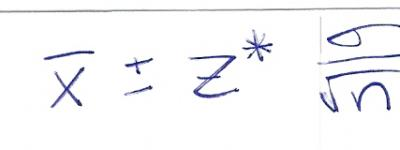Log In  |  Mobile View  |  Help
 Visiting CKSC 82 IPR Committee's Shares (account name: cksc_82)
(sample) Research Study Notes
 KEY (not always used, but used the same way) = Main Points       Key Words (get you points on tests)     Remember: these will save your life1/31 � Chapter 10Overview: Descriptive Statistics: Sum or Description of a populationInferential Statistics: Generalization of populations from samples Sample means (M) or proportions (P) allow us to infer a plausible value of a characteristic Confidence Intervals: A sample gives us a statistic (sample M or P). We are unable to say exactly what the population mean or proportion is. To get around this we say we have a confidence that our parameter lies with in an interval. This interval is centered around a sample proportion (a mean). Basically its a point estimate with a margin of error we tack on, a Confidence Interval. See book Definition for more info Confidence Level (C): For every Confidence Interval there is a C C is how much confidence" we have in our method. often choices for confidence levels are 90%, 95%, 99%. Remember: A Confidence Level is not a probability How to read a C: a C of 90% means "in the long run, 90% of the results from this method will capture the true value of the population parameter being estimated within the confidence interval." See book definition for more info Applied: Confidence Interval for a pop. mean (we are currently using z-intervals) To mix up a good C we work with three things SRS Pop. Mean (unknown) Standard deviation (won't have in reality, but have in current problems) In order to use z-intervals we MUST have #'s 1 and 3. A level C confidence interval of M is:Z* (actual symbol) = Upper Critical Value of S.N.D. (z-distributions!)Translated this means C is dervided from the SRS mean plus and minus Z* times the standard deviation  over the population2 Where as Z* is the positive value of the area to the left of one half the non confidence. A confidence of 99% leave 1% left over, half that is .005 - find that on the Z-Score box or witht he calc controls below and BAM you have your Z*! How do we find the Z*? just use {2nd} {Distr} -> {3}(invNorm)  this gives -> invNorm(area to left)Remember: book may write in interval notation: (98.1069,98.2991) Don't write this way! We write like this: 98.1009
None
Important
Creation date: Dec 23, 2009 11:33 pm     Last modified date: Dec 23, 2009 11:33 pm   Last visit date: Jul 18, 2019 1:40 am
Select a Color